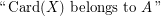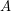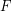# bounded tree width

## Complexity of QBF(Bounded Treewidth) ★★

Author(s): Moshe Y. Vardi

Question   What is the computational complexity of QBF(Bounded Treewidth)? Is it PSPACE-complete? In PTIME?

## Monadic second-order logic with cardinality predicates ★★

Author(s): Courcelle

The problem concerns the extension of Monadic Second Order Logic (over a binary relation representing the edge relation) with the following atomic formulas:

\item\itemwhereis a fixed recursive set of integers.

Let us fixand a closed formulain this language.

Conjecture   Is it true that the validity offor a graphof tree-width at mostcan be tested in polynomial time in the size of?